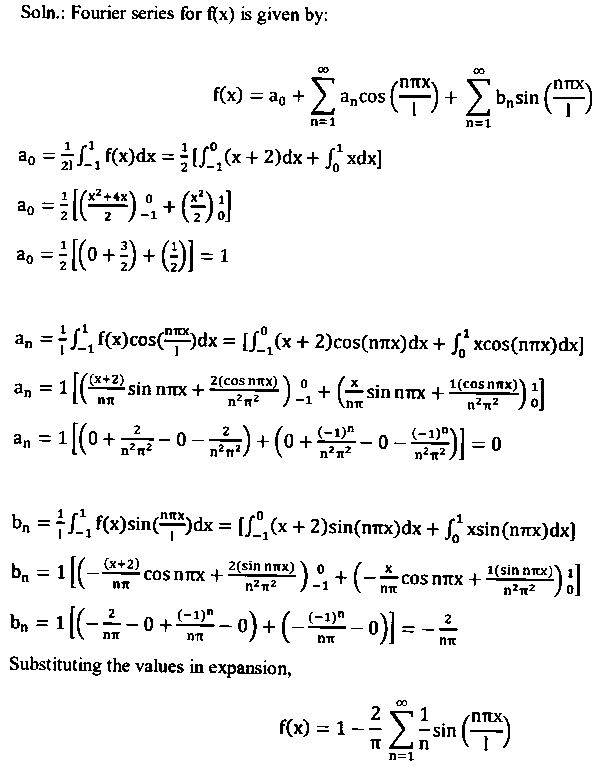# Find the Fourier Series of f(x)=x -1<x<0 & =x+2 0<x<1

more_vert

Find the Fourier Series of
f(x) = x -1 < x < 0
f(x) =x+2 0 < x < 1